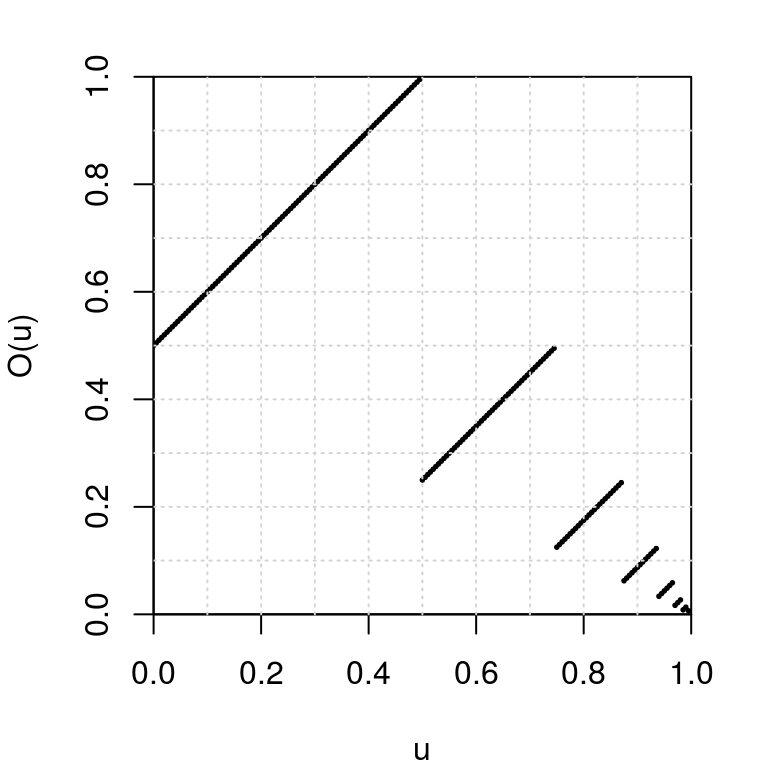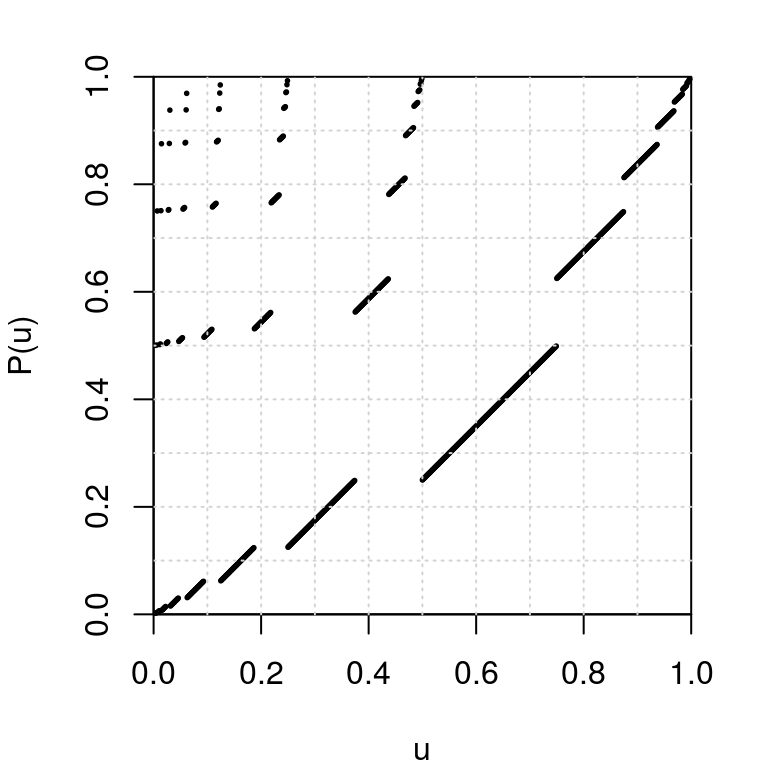2016-08-08
Source

We provide a function that computes the dyadic representation of a real number in the interval $[0,1]$. Then we give an implementation of two transformations of the set ${\{0,1\}}^\mathbb{N}$ which are well-known in ergodic theory: the dyadic odometer and the Pascal transformation. For each of these transformations, we plot the graph of the conjugate transformation of $[0,1]$ obtained by the dyadic representation.

Every real number $u \in [0,1]$ has a dyadic expansion (or binary expansion): $u = \frac{\epsilon_1}{2} + \frac{\epsilon_2}{2^2} + \frac{\epsilon_3}{2^3} + \ldots$ where $\epsilon_i=0$ or $1$. We say that the sequence $(\epsilon_1, \epsilon_2, \ldots)$ is the dyadic representation of $u$.

The num2dyadic function below returns the dyadic representation of $u \in [0,1]$.

out <- integer(nmax)
i <- j <- 0L
while(u>0 && i<nmax){
j <- 1L + max(0L,floor(-log2(u*(1+.Machine\$double.eps^.5))))
if(i+j <= nmax){
i <- i + j
out[i] <- 1L
u <- 2L^j*u - 1L
}else{
i <- nmax
}
}
return(out[1:i])
}

The dyadic2num function below does the reverse action:

Let us check that the dyadic representation of $0.75 = \frac{1}{2}+\frac{1}{4}$ is $(1,1)$:

##  1 1

The real number $u=0.2$ has the infinite periodic dyadic representation $(0, 0, 1, 1, 0, 0, 1, 1, \ldots)$. The num2dyadic function applied to $0.2$ returns only the first $54$ digits of its dyadic representation:

##   0 0 1 1 0 0 1 1 0 0 1 1 0 0 1 1 0 0 1 1 0 0 1 1 0 0 1 1 0 0 1 1 0 0 1
##  1 0 0 1 1 0 0 1 1 0 0 1 1 0 0 1 1 0 1
length(d)
##  54

But it makes no difference for R:

##  TRUE

The dyadic odometer is the transformation $O$ of the set ${\{0,1\}}^{\mathbb{N}}$ defined by $O(d) = d + (1, 0, 0, \ldots)$, where “$+$” is the addition $\bmod\, 2$ with carry to the right.

The odometer function below is an implementation of the dyadic odometer and its inverse (option image="backward").

odometer <- function(d, image=c("forward", "backward")){
image <- match.arg(image)
if(image=="forward"){
if(all(d==1L)){
d <- c(rep(0L, length(d)), 1L)
}else{
k <- which.min(d)
d[1:k] <- 1L-d[1:k]
}
}
if(image=="backward"){
if(all(d==0L)){
d <- c(rep(1L, length(d)), 0L)
}else{
k <- which.max(d)
d[1:k] <- 1L-d[1:k]
}
}
return(d)
}

Using the dyadic representation, the odometer also defines a map from the interval $[0,1]$ to itself. We plot its graph below:

par(mar=c(4,4,2,2))
u <- seq(0, 0.995, by=0.005)
plot(u, Ou, xlab="u", ylab="O(u)",
xlim=c(0,1), ylim=c(0,1),
pch=19, cex=.25,
pty="s", xaxs="i", yaxs="i")
grid(nx=10)The Pascal transformation

The Pascal transformation $P$ is defined for every $d \in {\{0,1\}}^{\mathbb{N}}$ except when $d=000\ldots$ or when $d$ has the form $d=0^i111\ldots$ ($i\geq 0$). Such a $d$ has the form $d= 0^m1^k10x$ where $m,k \geq 0$ and $x \in {\{0,1\}}^{\mathbb{N}}$, and then the image of $d$ by the Pascal transformation is $P(0^m1^k10x) = 1^k0^m01x.$ The case when $d=0^i111\ldots$ does not occur for us since we deal with finite sequences only. One naturally extends the Pascal transformation to include the case $d=000\ldots$ by setting $P(000\ldots) = 000\ldots$.

pascal <- function(d){
if(all(d==0L)) return(0L)
i <- which.max(d)
m1 <- i-1L
d0 <- c(d, 0L)
k1 <- which.min(d0[-(1:i)])-1L
begin <- c(rep(1L, k1), rep(0L, m1+1L), 1L)
if(length(d)==m1+k1+1L) d <- begin else d[1L:(m1+k1+2L)] <- begin
return(d)
}

By the dyadic representation, the Pascal transformation also defines a map from the interval $[0,1)$ to itself, whose graph is plotted below:

par(mar=c(4,4,2,2))
u <- seq(0, 1-1/2^10, by=1/2^10)